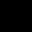# The radius of a semi circle is 6.3 cm then its perimeter is?

## 3 Answers on this Question

1.Akshay says:

perimeter of semi circle = (pi)r + 2r
= (3.14)(6.3)+(12.6)
= 32.382

2.Akshay says:

perimeter of semi circle = (pi)r
= (3.14)(6.3)
= 19.782 cm

1.Akshay says:

perimeter = pi(r) + 2r
= 19.782 + (12.6)
= 32.382 cm

Scroll to top